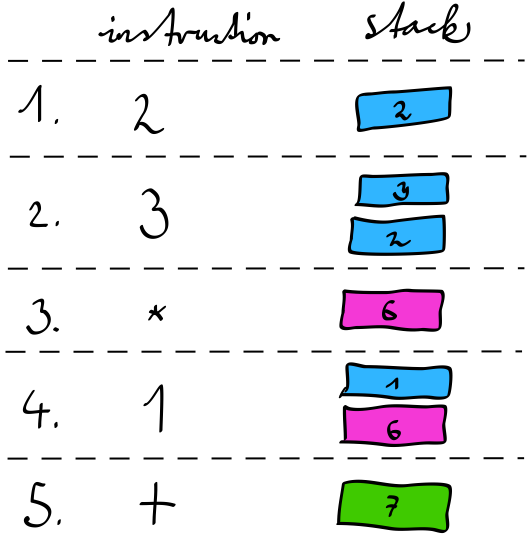Dec 2nd, 2013

# Stack Machines: RPN calculator

A very simple form of stack machine is a so-called RPN calculator. It is quite easy to understand and implement, and uses the same model that is used by most virtual machines. As such it’s very useful to study such a device in order to learn more about how VMs and actual computers work.

## Infix Notation

When describing mathematical formulae, it is common to use infix notation. Infix means that the operator is in between the two operands.

For example:

1 + 1

This notation has also been adopted by most popular programming languages1.

One of the downsides of infix notation is that certain expressions such as `1 + 2 * 3` are ambiguous and require special precedence rules and bracketing to be interpreted correctly.

Two alternate notations exist that do not have this limitation. Polish notation, which is known as prefix notation and — you guessed it — Reverse polish notation.

## Reverse Polish Notation

RPN stands for reverse polish notation. Another name for it is postfix notation. In simple terms, the operator comes after the operands.

For example:

1 1 +

This may look backwards at first2, but it is so much easier to implement a calculator for it.

The previous example of `1 + 2 * 3` can be unambiguously represented as:

2 3 * 1 +

The arity of `+` is fixed at 2: it takes exactly two arguments.

## Stack

It may not be obvious, but the algorithm for processing this stream of numbers and operations is based on a stack. There are two calculations happening. The first is `2 3 *` which produces a result.

But that result is not just thrown away, it is stored on a stack, allowing the next operation to use that data, which is exactly what the `... 1 +` does.

• A number just means push this number onto the stack.
• `+` means pop 2 elements from the stack, add them, push the result back.
• `*` does the same as `+` but for multiplication.

The symbols are instructions, the list above describes the instruction set.

The following drawing illustrates how the instructions affect the stack, which values get pushed onto and which ones get popped off of the stack.This also means that you can stack up a few values before actually processing them.

For example:

1 1 1 1 + + +

Will first push the number `1` four times. The first `+` will pop two of them, add them and push the resulting `2`. The second `+` will pop that `2` and another of the `1`s, add them, push `3`. The final `+` pops the `3`, pops the `1`, also adds them and pushes the final result, a `4`.

## Implementation

Now that we have the specs down, let’s implement this RPN calculator. It takes a bunch of instructions, executes them, and returns what is on top of the stack: the result.

Here it is, in all its glory3:

``````function execute(array \$ops)
{
\$stack = new \SplStack();

foreach (\$ops as \$op) {
if (is_numeric(\$op)) {
\$stack->push((int) \$op);
continue;
}

switch (\$op) {
case '+':
\$stack->push(\$stack->pop() + \$stack->pop());
break;
case '-':
\$n = \$stack->pop();
\$stack->push(\$stack->pop() - \$n);
break;
case '*':
\$stack->push(\$stack->pop() * \$stack->pop());
break;
case '/':
\$n = \$stack->pop();
\$stack->push(\$stack->pop() / \$n);
break;
case '%':
\$n = \$stack->pop();
\$stack->push(\$stack->pop() % \$n);
break;
default:
throw new \InvalidArgumentException(sprintf('Invalid operation: %s', \$op));
break;
}
}

return \$stack->top();
}
``````

As you can see, the implementation is trivial. It is simply a matter of looping over the instructions, pushing numbers onto the stack and switching on the individual instructions to do the right calculation.

A quick test run:

``````\$rpn = '2 3 * 1 +';
\$ops = explode(' ', \$rpn);
var_dump(execute(\$ops));
``````

Confirms that it works! It returns the value `7` as expected.

An RPN calculator is quite a trivial piece of software. And yet the mechanisms it uses emulate a subset of what an actual computer does.

Since this stack machine has no means of random access storage and no conditional branching instructions, it is still limited in its computational power. It is comparable to a pushdown automaton.

## Summary

• RPN is kind of backwards.
• Implementing an RPN calculator is easy.
• Hey, look! It’s a little computer!

1. There are some exceptions, including stack based languages such as Forth, Factor or Gershwin.

2. This implementation is roughly based on the RPN calculator from The C Programming Language by Brian W. Kernighan and Dennis M. Ritchie.